## Choose a Wavelet

There are two types of wavelet analysis: continuous and multiresolution. The type of wavelet analysis best suited for your work depends on what you want to do with the data. This topic focuses on 1-D data, but you can apply the same principles to 2-D data. To learn how to perform and interpret each type of analysis, see Practical Introduction to Time-Frequency Analysis Using the Continuous Wavelet Transform and Practical Introduction to Multiresolution Analysis.

### Time-Frequency Analysis

If your goal is to perform a detailed time-frequency analysis, choose the continuous wavelet transform (CWT). In terms of implementation, scales are discretized more finely in the CWT than in the discrete wavelet transform (DWT). For additional information, see Continuous and Discrete Wavelet Transforms.

#### Instantaneous Frequency

The CWT is superior to the short-time Fourier transform (STFT) for signals in which the instantaneous frequency grows rapidly. In the following figure, the instantaneous frequencies of the hyperbolic chirp are plotted as dashed lines in the spectrogram and CWT-derived scalogram. For additional information, see Time-Frequency Analysis and Continuous Wavelet Transform.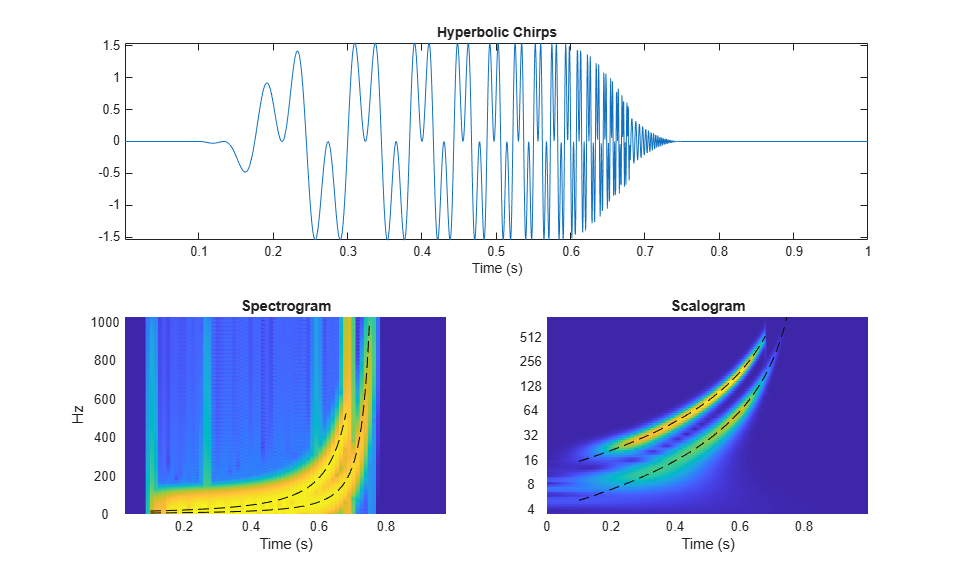#### Localizing Transients

The CWT is good at localizing transients in nonstationary signals. In the following figure, observe how well the wavelet coefficients align with the abrupt changes that occur in the signal. For additional information, see Practical Introduction to Time-Frequency Analysis Using the Continuous Wavelet Transform.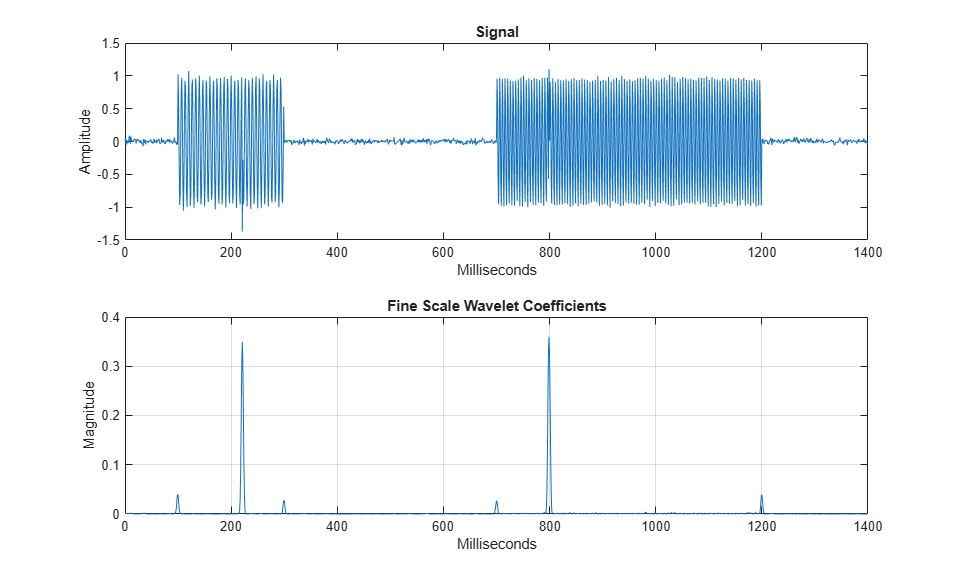#### Supported Wavelets

To obtain the continuous wavelet transform of your data, use `cwt` and `cwtfilterbank`. Both functions support the analytic wavelets listed in the following table. By default, `cwt` and `cwtfilterbank` use the generalized Morse wavelet family. This family is defined by two parameters. You can vary the parameters to recreate many commonly used wavelets. In the time-domain plots, the red line and blue lines are the real and imaginary parts, respectively, of the wavelet. The contour plots show the wavelet spread in time and frequency. For additional information, see Morse Wavelets and Generalized Morse and Analytic Morlet Wavelets.

WaveletFeaturesNameTime DomainTime-Frequency Domain
Generalized Morse WaveletCan vary two parameters to change time and frequency spread`"morse"` (default)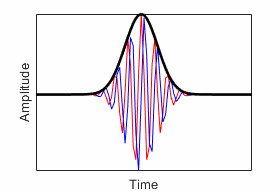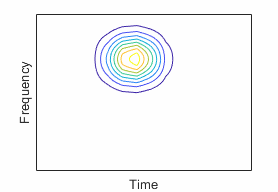Analytic Morlet (Gabor) WaveletEqual variance in time and frequency`"amor"`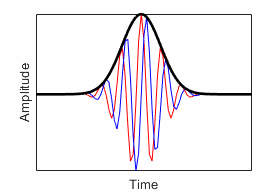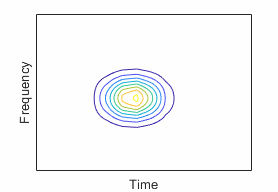Bump WaveletWider variance in time, narrower variance in frequency`"bump"`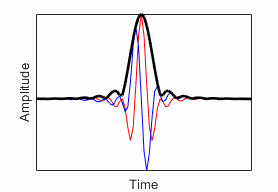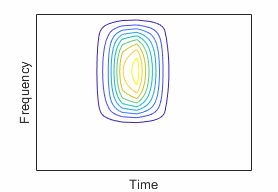All the wavelets in the table are analytic. Analytic wavelets are wavelets with one-sided spectra and are complex valued in the time domain. These wavelets are a good choice for obtaining a time-frequency analysis using the CWT. Because the wavelet coefficients are complex valued, the CWT provides phase information. `cwt` and `cwtfilterbank` support analytic and anti-analytic wavelets. For additional information, see CWT-Based Time-Frequency Analysis.

### Multiresolution Analysis

In a multiresolution analysis (MRA), you approximate a signal at progressively coarser scales while recording the differences between approximations at consecutive scales. You create the approximations and the differences by taking the discrete wavelet transform (DWT) of the signal. The DWT provides a sparse representation for many natural signals. Approximations are formed by comparing the signal with scaled and translated copies of a scaling function. Differences between consecutive scales, also known as details, are captured using scaled and translated copies of a wavelet. On a `log2` scale, the difference between consecutive scales is always 1. In the case of the CWT, differences between consecutive scales are finer.

When generating the MRA, you can either subsample (decimate) the approximation by a factor of 2 every time you increase the scale or not. Each option offers advantages and disadvantages. If you subsample, you end up with the same number of wavelet coefficients as the original signal. In the decimated DWT, translations are integer multiples of scale. For the nondecimated DWT, translations are integer shifts. A nondecimated DWT provides a redundant representation of the original data, but not as redundant as the CWT. Your application not only influences your choice of wavelet, but also which version of the DWT to use.

#### Energy Preservation

If preserving energy in the analysis stage is important, you must use an orthogonal wavelet. An orthogonal transform preserves energy. Consider using an orthogonal wavelet with compact support. Keep in mind that except for the Haar wavelet, orthogonal wavelets with compact support are not symmetric. The associated filters have nonlinear phase. This table lists supported orthogonal wavelets. See `wavemngr("read")` for all wavelet family names. To learn more about a specific family, including wavelets available in that family, use `waveinfo` and the family short name. For example, `waveinfo("db")`.

Orthogonal Wavelet Family
(Family Short Name)
Best-localized Daubechies (`"bl"`)

Compactly-supported wavelets similar to symlets; asymmetry of the symlets diminished in time by minimizing an additional second moment in time; scaling filter has N vanishing moments

`"blN"`, where N = 7, 9, and 10`blscalf`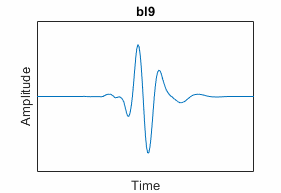Beylkin (`"beyl"`)Has 18 coefficients and has 3 vanishing moments.`"beyl"`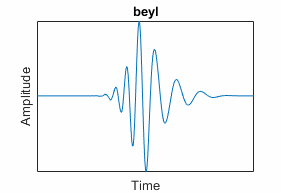Coiflets (`"coif"`)Scaling function and wavelets have same number of vanishing moments N`"coifN"`, where N = 1, 2, ..., 5`coifwavf`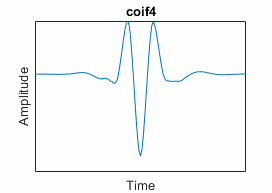Daubechies (`"db"`)Nonlinear phase; energy concentrated near the start of their support; highest number of vanishing moments N for a given support width`"dbN"`, where N = 1, 2, ..., 45`dbaux`, `dbwavf`, Extremal Phase Wavelet Coefficients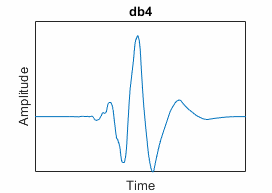Fejér-Korovkin (`"fk"`)Filters constructed to minimize the difference between a valid scaling filter and the ideal sinc lowpass filter; filters have N coefficients, are especially useful in discrete (decimated and undecimated) wavelet packet transforms.`"fkN"`, where N = 4, 6, 8, 14, 18, 22`fejerkorovkin`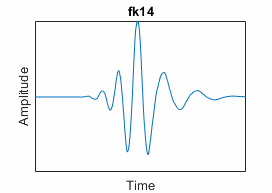Haar (`"haar"`)Symmetric; special case of Daubechies; useful for edge detection`"haar"`, or equivalently, `"db1"`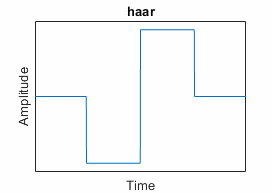Han linear-phase moments (`"han"`)Characterized by a specified order of sum rules SR and order of linear-phase moments LP`"hanSR.LP"`; to determine supported values, use `waveinfo` and the family short name`hanscalf`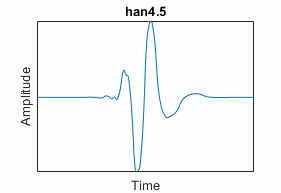Morris minimum-bandwidth (`"mb"`)Morris minimum-bandwidth orthogonal wavelets specified by the number of filter coefficients (taps) N and the level of the discrete wavelet transform L used in the optimization; do not pass default orthogonality checks in `isorthwfb``"mbN.L"`; to determine supported values, use `waveinfo` and the family short name`mbscalf`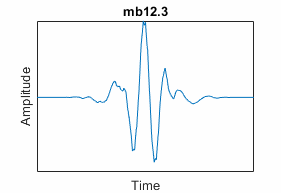Symlets (`"sym"`)Least asymmetric; nearly linear phase; N vanishing moments`"symN"` for N = 2, 3, ..., 45`symaux`, `symwavf`, Least Asymmetric Wavelet and Phase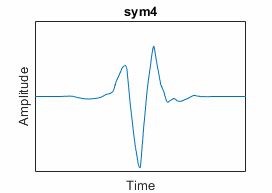Vaidyanathan (`"vaid"`)Has 24 coefficients; does not pass default orthogonality checks in `isorthwfb``"vaid"`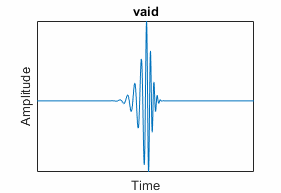Depending on how you address border distortions, the DWT might not conserve energy in the analysis stage. For more information, see Border Effects. The maximal overlap discrete wavelet transform `modwt` and maximal overlap discrete wavelet packet transform `modwpt` do conserve energy. The wavelet packet decomposition `dwpt` does not conserve energy.

#### Feature Detection

If you want to find closely spaced features, choose wavelets with smaller support, such as `haar`, `db2`, or `sym2`. The support of the wavelet should be small enough to separate the features of interest. Wavelets with larger support tend to have difficulty detecting closely spaced features. Using wavelets with large support can result in coefficients that do not distinguish individual features. In the following figure, the upper plot shows a signal with spikes. The lower plot shows the first-level MRA details of a maximal overlap DWT using the `haar` (thick blue lines) and `db6` (thick red lines) wavelets.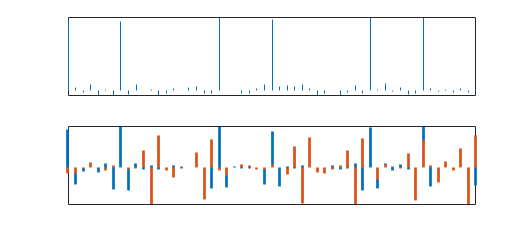If your data has sparsely spaced transients, you can use wavelets with larger support.

#### Analysis of Variance

If your goal is to conduct an analysis of variance, the maximal overlap discrete wavelet transform (MODWT) is suited for the task. The MODWT is a variation of the standard DWT.

• The MODWT conserves energy in the analysis stage.

• The MODWT partitions variance across scales. For examples, see Wavelet Analysis of Financial Data and Wavelet Changepoint Detection.

• The MODWT requires an orthogonal wavelet, such as a Daubechies wavelet or Symlet.

• The MODWT is a shift-invariant transform. Shifting the input data shifts the wavelet coefficients by an identical amount. The decimated DWT is not shift invariant. Shifting the input changes the coefficients and can redistribute energy across scales.

See `modwt`, `modwtmra`, and `modwtvar` for more information. See also Comparing MODWT and MODWTMRA.

#### Redundancy

Taking the decimated DWT, `wavedec`, of a signal using an orthonormal family of wavelets provides a minimally redundant representation of the signal. There is no overlap in wavelets within and across scales. The number of coefficients equals the number of signal samples. Minimally redundant representations are a good choice for compression, when you want to remove features that are not perceived.

The CWT of a signal provides a highly redundant representation of a signal. There is significant overlap between wavelets within and across scales. Also, given the fine discretization of the scales, the cost to compute the CWT and store the wavelet coefficients is significantly greater than the DWT. The MODWT `modwt` is also a redundant transform but the redundancy factor is usually significantly less than the CWT. Redundancy tends to reinforce signal characteristics and features you want to examine, such as frequency breaks or other transient events.

If your work requires representing a signal with minimal redundancy, use `wavedec`. If your work requires a redundant representation, use `modwt` or `modwpt`.

### Denoising

An orthogonal wavelet, such as a Symlet or Daubechies wavelet, is a good choice for denoising signals. A biorthogonal wavelet can also be good for image processing. Biorthogonal wavelet filters have linear phase which is very critical for image processing. Using a biorthogonal wavelet will not introduce visual distortions in the image.• An orthogonal transform does not color white noise. If white noise is provided as input to an orthogonal transform, the output is white noise. Performing a DWT with a biorthogonal wavelet colors white noise.

• An orthogonal transform preserves energy.

The `sym4` wavelet is the default wavelet used in `wdenoise` and Wavelet Signal Denoiser app. The `bior4.4` biorthogonal wavelet is the default wavelet in `wdenoise2`.

### Compression

If your work involves signal or image compression, consider using a biorthogonal wavelet. This table lists the supported biorthogonal wavelets with compact support.

Biorthogonal Wavelet Family
(Family Short Name)
FeaturesWavelet NameRepresentative
Biorthogonal Spline (`"bior"`)Compact support; symmetric filters; linear phase; specified by Nr and Nd, the number of vanishing moments for the reconstruction and decomposition filters respectively`"biorNr.Nd"`; see `waveinfo("bior")` for supported values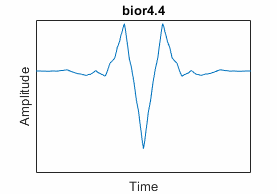Reverse Biorthogonal Spline (`"rbio"`)Compact support; symmetric filters; linear phase; specified by Nr and Nd, the number of vanishing moments for the reconstruction and decomposition filters respectively`"rbioNd.Nr"`; see `waveinfo("rbio")` for supported values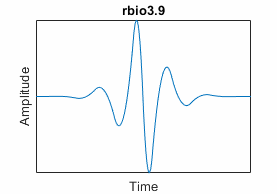Having two scaling function-wavelet pairs, one pair for analysis and another for synthesis, is useful for compression.

• Biorthogonal wavelet filters are symmetric and have linear phase. (See Least Asymmetric Wavelet and Phase.)

• The wavelets used for analysis can have many vanishing moments. A wavelet with N vanishing moments is orthogonal to polynomials of degree N-1. Using a wavelet with many vanishing moments results in fewer significant wavelet coefficients. Compression is improved.

• The dual wavelets used for synthesis can have better regularity. The reconstructed signal is smoother.

Using an analysis filter with fewer vanishing moments than a synthesis filter can adversely affect compression. For an example, see Image Reconstruction with Biorthogonal Wavelets.

When using biorthogonal wavelets, energy is not conserved at the analysis stage. See Orthogonal and Biorthogonal Filter Banks for additional information.

### General Considerations

Wavelets have properties that govern their behavior. Depending on what you want to do, some properties can be more important.

#### Orthogonality

If a wavelet is orthogonal, the wavelet transform preserves energy. Except for the Haar wavelet, no orthogonal wavelet with compact support is symmetric. The associated filter has nonlinear phase.

#### Vanishing Moments

A wavelet with N vanishing moments is orthogonal to polynomials of degree N−1. For an example, see Wavelets and Vanishing Moments. The number of vanishing moments and the oscillation of the wavelet have a loose relationship. As the number of vanishing moments grows, the greater the wavelet oscillates.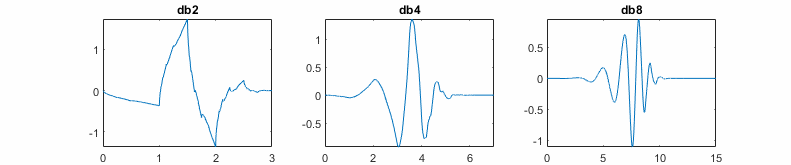The number of vanishing moments also affects the support of a wavelet. Daubechies proved that a wavelet with N vanishing moments must have a support of at least length 2N-1.

Names for many wavelets are derived from the number of vanishing moments. For example, `db6` is the Daubechies wavelet with six vanishing moments, and `sym3` is the Symlet with three vanishing moments. For coiflet wavelets, `coif3` is the coiflet with six vanishing moments. For Fejér-Korovkin wavelets, `fk8` is the Fejér-Korovkin wavelet with a length 8 filter. Biorthogonal wavelet names are derived from the number of vanishing moments the analysis wavelet and synthesis wavelet each have. For instance, `bior3.5` is the biorthogonal wavelet with three vanishing moments in the synthesis wavelet and five vanishing moments in the analysis wavelet. To learn more, see `waveinfo` and `wavemngr`.

If the number of vanishing moments N is equal to 1, 2, or 3, then `dbN` and `symN` are identical.

#### Regularity

Regularity is related to how many continuous derivatives a function has. Intuitively, regularity can be considered a measure of smoothness. To detect an abrupt change in the data, a wavelet must be sufficiently regular. For a wavelet to have N continuous derivatives, the wavelet must have at least N+1 vanishing moments. See Detecting Discontinuities and Breakdown Points for an example. If your data is relatively smooth with few transients, a more regular wavelet might be a better fit for your work.

 Daubechies, Ingrid. Ten Lectures on Wavelets. Society for Industrial and Applied Mathematics, 1992.

 Morris, Joel M, and Ravindra Peravali. “Minimum-Bandwidth Discrete-Time Wavelets.” Signal Processing 76, no. 2 (July 1999): 181–93. https://doi.org/10.1016/S0165-1684(99)00007-9.

 Doroslovački, M.L. “On the Least Asymmetric Wavelets.” IEEE Transactions on Signal Processing 46, no. 4 (April 1998): 1125–30. https://doi.org/10.1109/78.668562.

 Han, Bin. “Wavelet Filter Banks.” In Framelets and Wavelets: Algorithms, Analysis, and Applications, 92–98. Applied and Numerical Harmonic Analysis. Cham, Switzerland: Birkhäuser, 2017. https://doi.org/10.1007/978-3-319-68530-4_2.